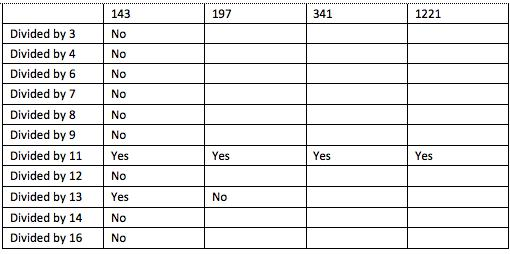#### You may also like### Consecutive Numbers

An investigation involving adding and subtracting sets of consecutive numbers. Lots to find out, lots to explore.### Roll These Dice

Roll two red dice and a green dice. Add the two numbers on the red dice and take away the number on the green. What are all the different possible answers?### Domino Square

Use the 'double-3 down' dominoes to make a square so that each side has eight dots.

# What's in the Box?

##### Age 7 to 11Challenge Level

We had a good number of solutions sent in with explanations as to how the answers were found. Ansh from Monkfield Park Primary School sent in the following:

For question 1) Answer is 8.
First of all I thought that 7 times 8 will be 56. Then I tried 3 times 8 and that also worked. Next I tried 13 times 8 that was 98 but that did not work so I tried 14 times 8 which was 112 and it was correct. Finally I divided 216 by 8 and ended up with 7.
So that's how I figured out that the multiplier is 8.
For question 2) Answer is 11
First of all I tried 3 and that did not work so I tried 6 and that did not work either. I also tried 7 and 9 but they both were wrong too. I knew it was not an even number so I skipped 2 and 10. Then I tried 11 and that worked.
So the first one was 13 times 11 = 143.
Next I divided 297 by 11 and the answer was 297.
After that I tried 341 divided by 11 and came up with 31.
Finally I did 1221 divided by 11 which gave me 111.
So the multiplier was 11.

Delaney at Mount Vernon School  Maine, U.S. said:

Question 1 Possible factors: 2, 4 or 8
Largest common factor: 8
$56\div2=28$,  $28\div2=14$,  $14\div2=7$
$24\div2=12$, $12\div2=6$, $6\div2=3$
$112\div2=56$, $56\div2=28$, $28\div2=14$
$216\div2=108$, $108\div2=54$, $54\div2=27$
After I got these answers there were no more common factors and I assumed that 7, 3, 14, and 27 were the original numbers. 8 is the largest common factor because $2^3$ or 2x2x2=8.

Question 2 Only possible factor: 11
None of the numbers were even in this question so I knew that no multiples of two would be a common factor.
143=110+33
297=220+77
1221=1100+121
341=330+11
After dividing into portions divisible by 11, I found the original numbers by dividing by 11.
$143\div11=13$
$341\div11=31$
$1221\div11=111$
$297\div11=27$
There were no more common factors, so I assumed those were the original numbers.

We had this submitted from Jerry at Dulwich College, Shanghai:

First I checked which numbers that 143 can be divided by.
I skipped the multiples of 5 because 143, 297, 341 and 1221 clearly wouldn't be able to be divided by multiples of 5. I first found out 143 can be divided by 11 and 13. But 297 cannot be divided by 13. So I kept working on 11 and all four numbers can be divided by 11. So the winner is 11!Well, altogether these have been very good responses to this challenge. Keep sending solutions to any other activity you have a go at.